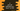# C program to print all Deficient numbers from 1 to 100## C program to print all Deficient numbers from 1 to 100:

In this post, we will learn how to print all deficient numbers from 1 to 100. The program will use a function to check if a number is deficient or not and by using a loop from 1 to 100, it will use this function to find and print all deficient numbers.

Let’s learn what is a deficient number and the algorithm that we will use in the program.

### What is a deficient number:

Deficient numbers are also known as defective numbers. It is a number whose sum of divisors of it is less than 2 * number. We can also find the proper divisors of a number to check if it is a defective number or not. For deficient numbers, the sum of proper divisors is less than the number.

For example, 21 is a deficient number. Its proper divisors are 1, 3 and 7. The sum is 1 + 3 + 7 = 11. It is smaller than 21. So, it is a deficient number.

18 is not a deficient number. Its proper divisors are 1, 2, 3, 6 and 9. The sum is 1 + 2 + 3 + 6 + 9 = 21. It is bigger than 18. So, it is not a deficient number.

### Algorithm to print all deficient numbers from 1 to 100:

The program will use the below algorithm to print all deficient numbers from 1 to 100:

• Run a loop from 1 to 100.
• On each iteration of this loop, check if the current value is deficient number or not. If it is, print the number.
• Use another method to check if a number is deficient number or not. This method will take a number as the parameter and return 1 if that number is deficient. Else, return 0.
• This method will take a number as the parameter.
• Initialize a variable as 0 to hold the sum of all proper divisors.
• By using a loop, it will run from 1 to number - 1 and find all proper divisors of that number. It will add the proper divisors to the sum variable.
• Once the loop ends, it will check if the sum is bigger than the number or not and based on that, it will return 1 or 0.

### Method 1: C program to print all deficient numbers from 1 to 100 using for loop:

Below is the complete C program:

``````#include <stdio.h>

int isDeficient(int n)
{
int sum = 0;

for (int i = 1; i < n; i++)
{
if (n % i == 0)
{
sum += i;
}
}

return sum < n;
}

int main()
{
printf("Deficient numbers within 1 to 100 are: ");

for (int i = 1; i <= 100; i++)
{
if (isDeficient(i))
{
printf("%d ", i);
}
}
printf("\n");

return 0;
}``````

Here,

• isDeficient method is used to check if a number is deficient or not.
• It takes a number as the parameter and returns 1 if it is a deficient number. Else, it returns 0.
• It initializes a variable sum as 0 to hold the sum of all proper divisors.
• It starts a for loop from 1 to number - 1 and it finds all proper divisors within this range. If any proper divisor is found it adds this value to the sum variable.
• It compares the value of sum with n and returns 1 or 0. If sum is smaller than n, it returns 1. Else, it returns 0.
• The main method runs first. It uses a for loop that runs from 1 to 100 and for each value, it calls the isDeficient method to check if it is a deficient number or not.
• If yes, it prints its value.

### Output:

If you run the above program, it will give the below output:

``Deficient numbers within 1 to 100 are: 1 2 3 4 5 7 8 9 10 11 13 14 15 16 17 19 21 22 23 25 26 27 29 31 32 33 34 35 37 38 39 41 43 44 45 46 47 49 50 51 52 53 55 57 58 59 61 62 63 64 65 67 68 69 71 73 74 75 76 77 79 81 82 83 85 86 87 89 91 92 93 94 95 97 98 99 ``

### Method 2: C program to print all deficient numbers from 1 to 100 using while loop:

We can also use a while loop instead of a for loop in the above program. It will work in a similar way.

Below is the complete program:

``````#include <stdio.h>

int isDeficient(int n)
{
int sum = 0, i = 1;

while (i < n)
{
if (n % i == 0)
{
sum += i;
}
i++;
}

return sum < n;
}

int main()
{
int i = 1;
printf("Deficient numbers within 1 to 100 are: ");

while (i <= 100)
{
if (isDeficient(i))
{
printf("%d ", i);
}
i++;
}
printf("\n");

return 0;
}``````

If you run this program, it will print:

``Deficient numbers within 1 to 100 are: 1 2 3 4 5 7 8 9 10 11 13 14 15 16 17 19 21 22 23 25 26 27 29 31 32 33 34 35 37 38 39 41 43 44 45 46 47 49 50 51 52 53 55 57 58 59 61 62 63 64 65 67 68 69 71 73 74 75 76 77 79 81 82 83 85 86 87 89 91 92 93 94 95 97 98 99 ``